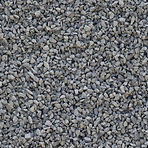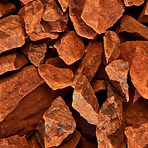## OUR PRODUCTS

Company RUDOMAIN produces iron ores of the following subspecies: sinter ore, blast furnace ore, raw ore and iron ore.

Products meet the requirements of DSTU 3704.#### SINTER ORE  SR-1

Fe≥60%

Mass fraction of total iron,%, is not less than 60.0

Mass fraction of silicon dioxide,%, is not more than 14.0

Mass fraction of moisture,%, is not more than 8.0

Fineness (maximum piece size), mm, not more than 20.0

Mass fraction of the control class of fineness +10 mm,%, is not more than 10.0

Mass fraction of phosphorus,%, is not more than 0.01

Mass fraction of sulfur,%, is not more than 0.01#### SR-2

Fe≥55%

Mass fraction of total iron,%, is not less than 55.0

Mass fraction of silicon dioxide,%, is not more than 21.0

Mass fraction of moisture,%, is not more than 8.0

Fineness (maximum piece size), mm, not more than 20.0

Mass fraction of the control class of fineness +10 mm,%, is not more than 10.0

Mass fraction of phosphorus,%, is not more than 0.01

Mass fraction of sulfur,%, is not more than 0.01#### SR-3

Fe≥50%

Mass fraction of total iron,%, is not less than 50.0

Mass fraction of silicon dioxide,%, is not more than 24.0

Mass fraction of moisture,%, is not more than 8.0

Fineness (maximum piece size), mm, not more than 20.0

Mass fraction of the control class of fineness +10 mm,%, is not more than 18.0

Mass fraction of phosphorus,%, is not more than 0.01

Mass fraction of sulfur,%, is not more than 0.01#### BO-1

Fe≥50%

Mass fraction of total iron,%, is not less than 50.0

Mass fraction of Fe2О3,% , is not less than –

Deviation from the mass fraction of iron,%, is ± 1

Mass fraction of silicon dioxide,%, is not more than 26.0

Mass fraction of moisture,%, is not more than 4.0

Fineness (maximum piece size), mm, not more than 100.0

Mass fraction of the control class of fineness 0-10 mm,%, is not more than – 20

Mass fraction of the control class of fineness +10 mm,%, is not more than –

Mass fraction of phosphorus,%, is not more than 0.01

Mass fraction of sulfur,%, is not more than 0.01#### BO-2

Fe≥47%

Mass fraction of total iron,%, is not less than 47.0

Mass fraction of Fe2О3,% , is not less than –

Deviation from the mass fraction of iron,%, is ± 1

Mass fraction of silicon dioxide,%, is not more than 31.0

Mass fraction of moisture,%, is not more than 4.0

Fineness (maximum piece size), mm, not more than 100.0

Mass fraction of the control class of fineness 0-10 mm,%, is not more than – 20

Mass fraction of the control class of fineness +10 mm,%, is not more than –

Mass fraction of phosphorus,%, is not more than 0.01

Mass fraction of sulfur,%, is not more than 0.01#### CO

Fe≥46%

Mass fraction of total iron,%, is not less than 46.0

Mass fraction of Fe2О3,% , is not less than – 65

Deviation from the mass fraction of iron,%, is ± 1

Mass fraction of silicon dioxide,%, is not more than 25.0

Mass fraction of moisture,%, is not more than 8.0

Fineness (maximum piece size), mm, not more than 20.0

Mass fraction of the control class of fineness 0-10 mm,%, is not more than – 80

Mass fraction of the control class of fineness +10 mm,%, is not more than – 20

Mass fraction of phosphorus,%, is not more than 0.01

Mass fraction of sulfur,%, is not more than 0.01#### CO-1

Fe≥42%

Mass fraction of total iron,%, is not less than 42.0

Mass fraction of Fe2О3,% , is not less than – 60

Deviation from the mass fraction of iron,%, is ±1

Mass fraction of silicon dioxide,%, is not more than 28.0

Mass fraction of moisture,%, is not more than 8.0

Fineness (maximum piece size), mm, not more than 20.0

Mass fraction of the control class of fineness 0-10 mm,%, is not more than – 80

Mass fraction of the control class of fineness +10 mm,%, is not more than – 20

Mass fraction of phosphorus,%, is not more than 0.01

Mass fraction of sulfur,%, is not more than 0.01#### CO-2

Fe≥40%

Mass fraction of total iron,%, is not less than 40.0

Mass fraction of Fe2О3,% , is not less than – 56

Deviation from the mass fraction of iron,%, is ±1

Mass fraction of silicon dioxide,%, is not more than 33.0

Mass fraction of moisture,%, is not more than 8.0

Fineness (maximum piece size), mm, not more than 20.0

Mass fraction of the control class of fineness 0-10 mm,%, is not more than – 80

Mass fraction of the control class of fineness +10 mm,%, is not more than – 20

Mass fraction of phosphorus,%, is not more than 0.01

Mass fraction of sulfur,%, is not more than 0.01#### CO-3

Fe≥38%

Mass fraction of total iron,%, is not less than 38.0

Mass fraction of Fe2О3,% , is not less than – 52

Deviation from the mass fraction of iron,%, is ±1

Mass fraction of silicon dioxide,%, is not more than 38.0

Mass fraction of moisture,%, is not more than 8.0

Fineness (maximum piece size), mm, not more than 20.0

Mass fraction of the control class of fineness 0-10 mm,%, is not more than – 80

Mass fraction of the control class of fineness +10 mm,%, is not more than – 20

Mass fraction of phosphorus,%, is not more than 0.01

Mass fraction of sulfur,%, is not more than 0.01#### LOW-GRADE ORE RO

Fe≥39%

Mass fraction of total iron,%, is not less than 39.0

Deviation from the mass fraction of iron,%, is ±2

Mass fraction of moisture,%, is not more than 8.0

Fineness (maximum piece size), mm, not more than 5000.0

Mass fraction of phosphorus,%, is not more than 0.01

Mass fraction of sulfur,%, is not more than 0.01#### RO-1

Fe≥44%

Mass fraction of total iron,%, is not less than 44.0

Deviation from the mass fraction of iron,%, is ±2

Mass fraction of moisture,%, is not more than 8.0

Fineness (maximum piece size), mm, not more than 5000.0

Mass fraction of phosphorus,%, is not more than 0.01

Mass fraction of sulfur,%, is not more than 0.01#### IRON ORE IO-1.1

Fe≥54%

Mass fraction of total iron,%, is not less than 44.0

Deviation from the mass fraction of iron,%, is ±2

Mass fraction of moisture,%, is not more than 8.0

Fineness (maximum piece size), mm, not more than 5000.0

Mass fraction of phosphorus,%, is not more than 0.01

Mass fraction of sulfur,%, is not more than 0.01#### IRON ORE IO-2.2

Fe≥59%

Mass fraction of total iron,%, is not less than 59.0

Deviation from the mass fraction of iron,%, is ±2

Mass fraction of moisture,%, is not more than 8.0

Fineness (maximum piece size), mm, not more than 5000.0

Mass fraction of phosphorus,%, is not more than 0.01

Mass fraction of sulfur,%, is not more than 0.01